Heinrich Hartmann

# Anomaly Detection

Written on 2014-07-10

## Definition of Time Series

Definition $\def\KM{\mathcal{M}} \def\IY{\mathbb{Y}}$

A time series (instance) $y$ is a sequence of real numbers:

A time series model $\IY$ is a sequence of random variables:

Examples $\def\eps{\epsilon}$ $\def\KN{\mathcal{N}}$ $\def\IR{\mathbb{R}}$

1. Let $\eps_t \sim \KN(0,1)$ be independent standard-normally distributed. Then the time series model with $\IY_t = \eps_t$ is called standard-white noise.

2. Let $b \in \IR$ be a real number, then $\IY_t = b + \eps_t$ is a time series model with constant expectation $E[\IY_t] = b$

3. Let $b,m \in \IR$ be real numbers, then $\IY_t = b + m t + \eps_t$ is a time series with affine-linear expectations $E[\IY_t] = b + m t$

Remark

For a given time series $y$ and model $\IY$ we get a probability for the occurencye of $y$:

In case the model has a joint probability density function $p_\IY$ we get a probability density for the time series $y$: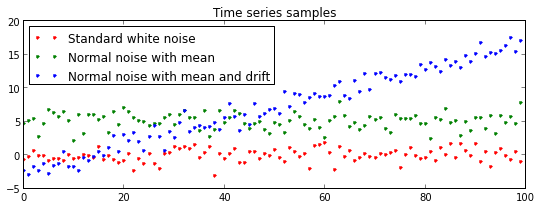## Parameter Estimation

Definition Let $\IY_\theta$ for $\theta \in \Theta$ be a family of time series models, and let $y$ be a time series instance. We write $P_\theta$ and $p_\theta$ for $P_{\IY_\theta}$ and $p_{\IY_\theta}$.

The maximum likelihood estimator (MLE) (cf. wikipedia) for $y$ in $\Theta$ is defined as

In case the model has a density function $p$ we set

Note that the maximum likelyhood estimater does not need to exists, nor is it unique if it exists.

Example

1. Let $\Theta=\IR$ and $\theta = b$ with model $\IY_t = b + \eps_t$, then

The value $p_b$ is maximal if the sum of squares $\sum_t (b - y_t)^2$ is mininmal. Threfore

1. Similarly, for the model $\IY_t = b + m t + \eps$ and $\theta=(b,m)$ the MLE minimizes the following sum of squares

This problem is quivalent to Linear Regression.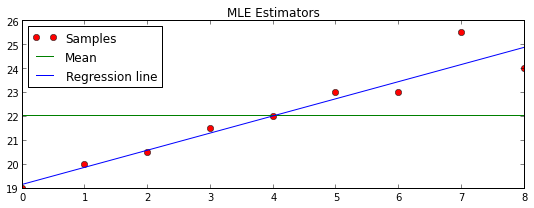# Hypothesis Testing

Let $\IY_\theta$ and $\IY_{\theta'}$ be two families of models, indexed by $\theta \in \Theta_0, \theta' \in \Theta_1$ and $y$ a time series instance. We consider the following statements:

• $H_0:$ The sample $y$ was drawn from a model in $\{\IY_\theta\}$
• $H_1:$ The sample $y$ was drawn from a model in $\{\IY_{\theta'} \}$

In order to decide between those statements, we consider their likelihood ratio.

Definition

We define the Likelihood ratio $\Lambda$ to be

or, in the continues case

We define the Log-likelihood ratio as $\lambda = log(\Lambda)$.

For a given confidence level $\alpha \geq 1$ we accept the Hypythesis $H_1$ if $\Lambda > \alpha$.

Example $\def\half{\frac{1}{2}}$

Take $% $, a time series $y$ and $\theta = b_0, \theta' = b_1$. To test the hypothesis

• $H_0$: The instance $y$ was drawn from $\IY_t = b_0 + \eps_t$
• $H_1$: The instance $y$ was drawn from $\IY_t = b_1 + \eps_t$

we calculate the likelihood ratios as:

where $\bar{b} = \half (b_0 + b_1)$ is the average of $b_0, b_1$, the variable $\delta = \half(b_1 - b_0)$ is half the distance between $b_1$ and $b_0$ and $\bar{y} = \frac{1}{T} \sum_t y_t$ is the sample mean. For the second step we used the following simple identity:

Note, that $\lambda = 0$ if $\bar{b} = \bar{y}$. And $\lambda > 0$ if $\bar{y} - \bar{b} > 0$, i.e. $\bar{y}$ is closer to $b_1$ than to $b_0$.

We accept the Hypothesis $H_1$ if $\lambda > log(\alpha)$, which is equivalent to: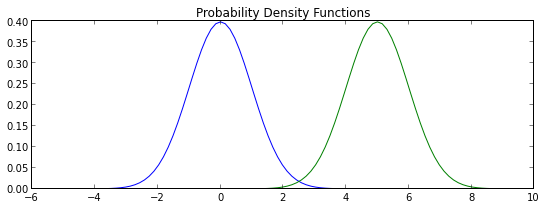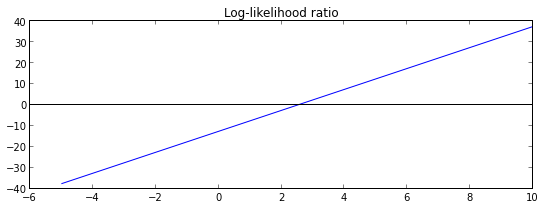l2 == l ? True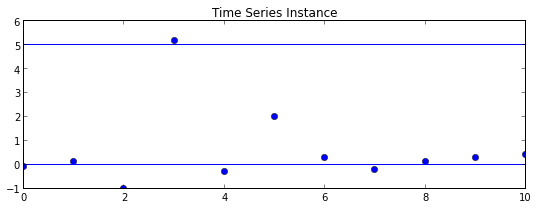Hypothesis H_1 accepted: False


Log likelihood ratio $\lambda=-103.5$ for plotted time series Y

# Test for changes in mean

For given $% $ we consider the hypotheses:

• $H_0$: Constant mean model $\IY_t = b_0 + \eps_t$

• $H_1$: Change in mean at a time $k \in \{ 0, \dots, T\}$: $\IY_t = b_0 + \eps_t, \text{ for } t \leq k$ $\IY_t = b_1 + \eps_t, \text{ for } t > k$

For an instance $y$ we calculate the log-likelihood ration to be $\lambda = \max_k \{ \lambda_k \}$ and using the notation from the last example we get

We introduce the notation $S_k^l = 2 \delta \sum_{t=k}^l (y_t - \bar{b})$ so that we can write

The total log likelihood ratio is computed by explicit maximization:

Note, that $\lambda \geq 0$, since $\min \leq S_1^T$.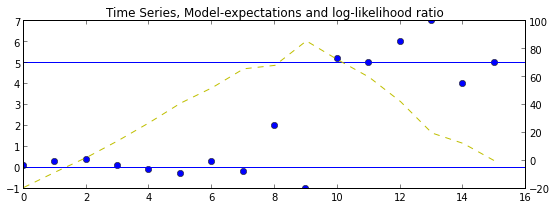## Online Variant

It turns out, that there is a simple recursion, which allows us to compute the likelyhood ratio $\lambda(T+1)$ for an instance $y_1,\dots,y_T$ of length $T+1$ from the knowledge of $\lambda(T)$ for the instance $(y_1,\dots,y_T)$ of length $T$.

Indeed, we have $\lambda(T+1) = S_1^{T+1} - \min_{k=1,\dots,T+1} S_1^{k}.$ Note, that $S_1^{T+1} = S_1^T + 2 \delta (y_t - \bar{b})$.

The minimum-term we have

$% $ In case $(A)$ we have $\lambda(T+1) = 0$ and in case $(B):$ $\lambda(T+1) = \lambda(T) + 2 \delta (y_{T+1} - \bar{b}).$

Since we always have $\lambda(T) \geq 0$, we get the total recursion:

Set $g(T) := \lambda(T) / (2 \delta)$ then we get the recursion:

which is known as the CUSUM method.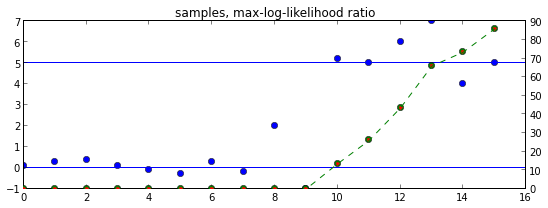View the version history of this post on GitHub.
Comments have been disabled until the dust around the GDPR settled.